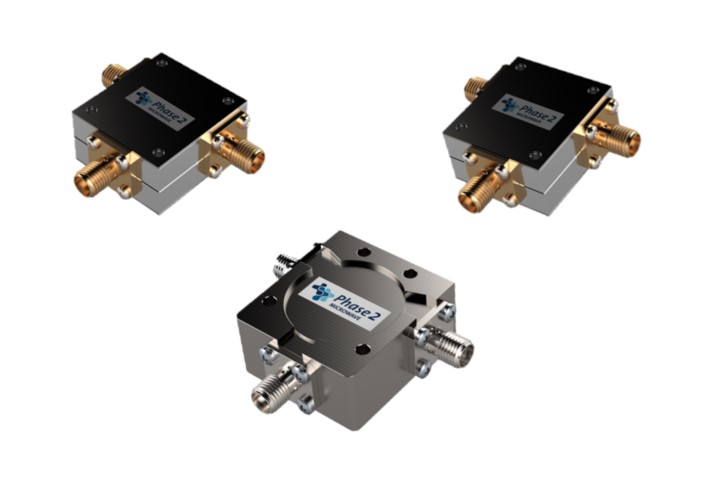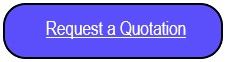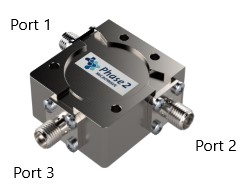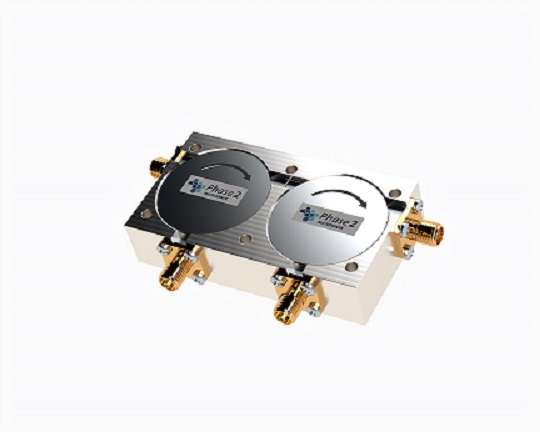Product OverviewPhase 2 Microwave Ltd offer a range of standard single junction Coaxial Circulators and Isolators with SMA Female connectors, covering many commonly used bands ranging from 700MHz to 6GHz.

These  offer a cost effective solution for many industry applications and can be supplied in small, medium and large quantities and are shown in the tables below.The standard direction of circulation is Port 1 to Port 2 as shown in the following diagram belowHowever if you require this to be reversed please specify at time of order

Circulators 3 - 6GHz

 Part Number Frequency (GHz) Power (W) Insertion Loss (dB) Isolation (dB) VSWR Size (mm) Connector Datasheet 006472 2.70-6.20 100 <=0.8 >=16 <=1.25 25.4x28.0x14.0 SMA Female 006473 3.00-6.00 100 <=0.6 >=18 <=1.35 25.4x28.0x14.0 SMA Female

Circulators 700MHz - 3GHz

 Part Number Frequency (GHz) Power (W) Insertion Loss (dB) Isolation (dB VSWR Size   (mm) Connector Datasheet 006482 0.70-1.30 100 <=0.6 >=18. <=1.30 30.0x33.0x15.0 SMA Female006480 0.70-0.90 100 <=0.4 >=20 <=1.25 30.0x33.0x15.0 SMA Female 006483 0.80-1.00 100 <=0.4 >=20 <=1.Pa25 30.0x33.0x15.0 SMA Female 006481 0.89-0.96 100 <=0.3 >=23 <=1.20 30.0x33.0x15.0 SMA Female 006474 0.95-1.45 100 <=0.6 >=15 <=1.45 30.0x33.0x15.0 SMA Female 006476 0.96-1.215 100 <=0.3 >=21 <=1.2 30.0x33.0x15.0 SMA Female 006475 0.96-1.26 100 <=0.45 >=20 <=1.25 30.0x33.0x15.0 SMA Female 006477 1.03-1.09 100 <=0.25 >=25 <=1.15 30.0x33.0x15.0 SMA Female 006478 1.29-1.31 100 <=0.25 >=25 <=1.15 30.0x33.0x15.0 SMA Female 006479 1.35-1.85 100 <=0.5 >=20 <=1.25 30.0x33.0x15.0 SMA Female 006484 1.40-1.70 100 <=0.45 >=20 <=1.25 30.0x33.0x15.0 SMA Female 006485 1.5-3.0 100 <=0.6 >=17 <=1.35 30.0x33.0x15.0 SMA Female 006486 1.70-2.30 100 <=0.5 >=18 <=1.30 30.0x33.0x15.0 SMA Female 006487 1.90-2.20 100 <=0.4 >=20 <=1.25 30.0x33.0x15.0 SMA Female 006488 2.10-2.70 100 <=0.5 >=18 <=1.30 30.0x33.0x15.0 SMA Female 006489 2.20-2.40 100 <=0.3 >=23 <=1.2 30.0x33.0x15.0 SMA Female 006490 2.30-2.70 100 <=0.4 >=20 <=1.25 30.0x33.0x15.0 SMA Female 006491 2.50-2.70 100 <=0.3 >=23 <=1.2 30.0x33.0x15.0 SMA Female 006492 2.70-2.90 100 <=0.3 >=23 <=1.2 30.0x33.0x15.0 SMA Female 006493 2.80-3.00 100 <=0.3 >=23 <=1.2 30.0x33.0x15.0 SMA Female

. Step files for the Circulators listed above are available on request.

All of the above are available in an Isolator format with an integral termination normally on port 3, or they can be configured as double junction devices if more isoation is required.To get a step file or discuss these options please ,